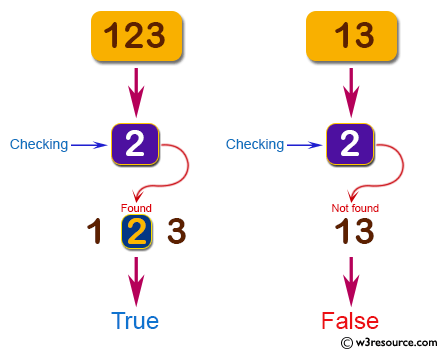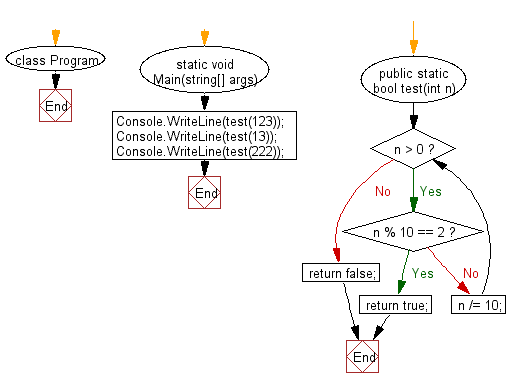﻿ C# - Return true if a positive integer contains 2# C# Sharp Basic Algorithm Exercises: Check a positive integer and return true if it contains a number 2

## C# Sharp Basic Algorithm: Exercise-139 with Solution

Write a C# Sharp program to check a positive integer and return true if it contains a number 2.

Pictorial Presentation:Sample Solution:

C# Sharp Code:

``````using System;
using System.Collections;
using System.Collections.Generic;
using System.Linq;
using System.Text;
namespace exercises
{
class Program
{
static void Main(string[] args)
{
Console.WriteLine(test(123));
Console.WriteLine(test(13));
Console.WriteLine(test(222));
}
public static bool test(int n)
{
while (n > 0)
{
if (n % 10 == 2) return true;
n /= 10;
}
return false;
}
}
}
```
```

Sample Output:

```True
False
True
```

Flowchart:C# Sharp Code Editor:

Improve this sample solution and post your code through Disqus

What is the difficulty level of this exercise?

Test your Programming skills with w3resource's quiz.

﻿